Agnes Grey

Plaintext for Anne Brontë's "Agnes Grey"

Examples

Basic Examples

Retrieve the ResourceObject:

 In:=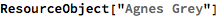Out=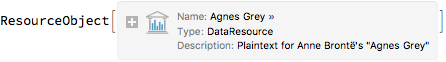View the data:

 In:=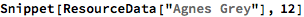Out=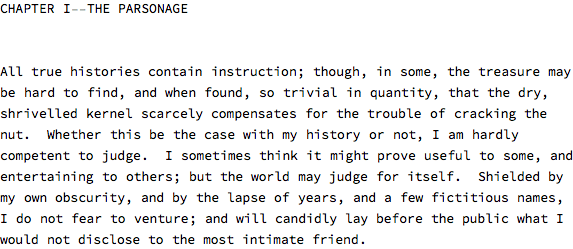Visualization

Generate a word cloud:

 In:=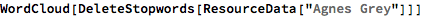Out=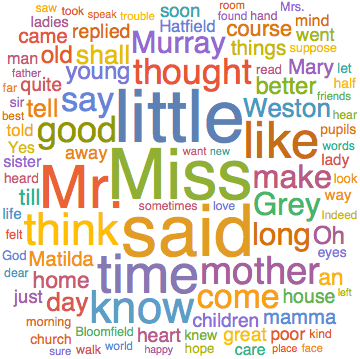Show the distribution of word lengths:

 In:=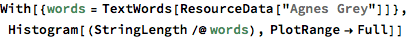Out=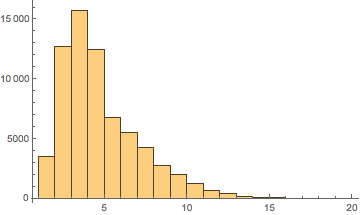Wolfram Research, "Agnes Grey" from the Wolfram Data Repository (2016) https://doi.org/10.24097/wolfram.81659.data